# 1. Using the given information answer the following questions with corresponding answers. (a) Serine is a diprotic acid...

1. Using the given information answer the following questions with corresponding answers.

(a)

Serine is a diprotic acid (Ka1 = 6.17× 10-3 and Ka2 = 7.08× 10-10) that can have three different forms in solution: H2S+, HS, and S- (Note: S is not sulfur in these formulas). What would be the concentration of H2S+ in the solution of 0.105M Na+S-?

8.29× 10-8 M

1.62× 10-12 M

9.62× 10-9 M

4.15× 10-11 M

7.08× 10-10 M

(b)

Serine is a diprotic acid (Ka1 = 6.17× 10-3 and Ka2 = 7.08× 10-10) that can have three different forms in solution: H2S+, HS, and S- (Note: S is not sulfur in these formulas). What would be the isoelectric point of 0.010M serine in solution?

6.925

5.680

8.126

7.000

4.152

(c)

Lysine is a triprotic acid (Ka1 = 6.61× 10-3, Ka2 = 1.12× 10-9, and Ka3 = 2.95×10-11) that can have four different forms in solution: H3L2+, H2L+, HL, and L-. What would be the pH of the solution containing 0.080M H3L+?

1.70

3.08

2.39

2.05

0.73

(d)

Lysine is a triprotic acid (Ka1 = 6.61× 10-3, Ka2 = 1.12× 10-9, and Ka3 = 2.95×10-11) that can have four different forms in solution: H3L2+, H2L+, HL, and L-. What would be the concentration of L- in the solution of 0.080M H3L+?

1.1× 10-9 M

2.9× 10-11 M

1.7× 10-18 M

6.7× 10-16 M

4.7× 10-17 M

We were unable to transcribe this image
We were unable to transcribe this image
We were unable to transcribe this image
We were unable to transcribe this image
We were unable to transcribe this image
We were unable to transcribe this image
We were unable to transcribe this image
We were unable to transcribe this image
We were unable to transcribe this image
We were unable to transcribe this image
We were unable to transcribe this image
We were unable to transcribe this image
We were unable to transcribe this image
We were unable to transcribe this image
We were unable to transcribe this image
We were unable to transcribe this image
We were unable to transcribe this image
We were unable to transcribe this image
We were unable to transcribe this image
We were unable to transcribe this image

Q1(a). Correct answer is : 1.62 x 10-12

Q1(b). Correct answer is : 5.680

Q1(c). Correct answer is : 1.70

Q1(d). Correct answer is : 2.9 x 10-11 M

Explanation

Q1. The hydrolysis equation for S- is

S- (aq) + H2O (l) HS (aq) + OH- : Kb1

HS (aq) + H2O (l) H2S+ (aq) + OH- : Kb2

Kb2 = (Kw) / (Ka1)

Kb2 = (1.0 x 10-14) / (6.17 x 10-3)

Kb2 = 1.62 x 10-12

For second hydrolysis, concentration of H2S+ is almost equal to Kb2

[H2S+] = Kb2 = 1.62 x 10-12

#### Earn Coin

Coins can be redeemed for fabulous gifts.

Similar Homework Help Questions
• ### 1. Using the given information answer the following questions with corresponding answers. (a) Tryptophan is a...

1. Using the given information answer the following questions with corresponding answers. (a) Tryptophan is a diprotic acid (Ka1 = 1.48 × 10-3 and Ka2 = 4.07 × 10-10) that can have three different form in solution: H2T+, HT, and T-. What would be the pH of the solution containing 0.045M HT? Group of answer choices 6.86 6.12 7.43 12.23 7.06 (b) Tryptophan is a diprotic acid (Ka1 = 1.48 × 10-3 and Ka2 = 4.07 × 10-10) that can...

• ### Use the given information to answer the following questions with corresponding answers. (a) Consider the following disso...

Use the given information to answer the following questions with corresponding answers. (a) Consider the following dissociation reactions of glycine that can have three different forms in solution: H2G+, HG, or G-. : H2G+  ⟺   HG + H+ Ka1 = 4.6 × 10-3 HG  ⟺   G- + H+   Ka2 = 2.5 × 10-10 Determine the dissociation constant for G- + H2O  ⇔   HG + OH-. Group of answer choices 4.0 ×× 10-9 4.0 ×× 10-5 2.2 ×× 10-12 3.5 ×× 10-6 2.9 ××...

• ### 1 A) Which of the following pairs of solutions when mixed will form a buffer solution?...

1 A) Which of the following pairs of solutions when mixed will form a buffer solution? Group of answer choices H2SO4 and Ca(OH)2 NH3 and NH4Br NaNO3 and HNO3 KOH and KNO2 B)Phosphoric acid, H3PO4, is a triprotic acid with the following Ka values: Ka1 = 7.5 x 10-3; Ka2 = 6.2 x 10-8; Ka3 = 3.6 x 10-13 In a solution of 0.15 M H3PO4, which species will be most abundant? H3O+ H3PO4 HPO42- H2PO4-

• ### Which of the following would be considered the most acidic amino acid? alanine serine leucine glycine...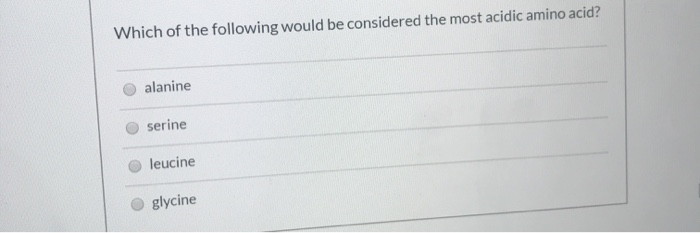Which of the following would be considered the most acidic amino acid? alanine serine leucine glycine We were unable to transcribe this imageQuestion 3 2 pts When placed in water, proteins fold into discrete three dimensional shapes. Which amino acid is least likely to be found on the surface of a protein in water? lysine serine aspartic acid valine Describe how you would synthesize the dipeptide Leu-Gly. HTML Editor I EE xx, BIUA T T12pt

• ### We were unable to transcribe this imageUse the References The acid ionization constant for In(H20) +...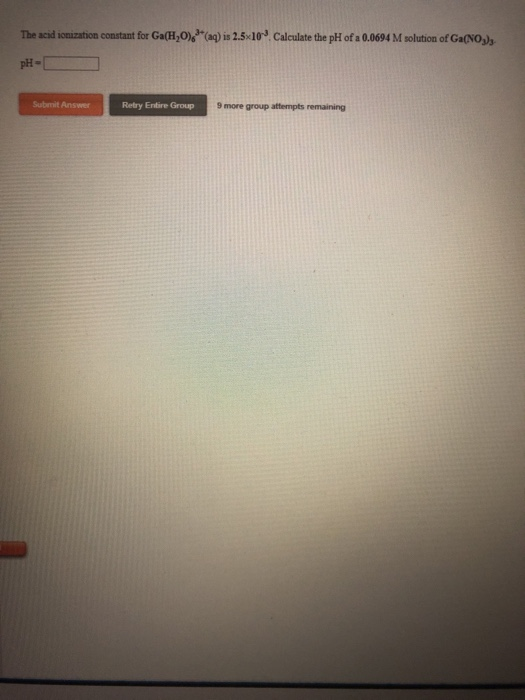We were unable to transcribe this imageUse the References The acid ionization constant for In(H20) + (aq) is 1.0*10* Calculate the pH of a 0.0173 M solution of In(NO3)3 pH- Submit Answer Retry Entire Group

• ### please answer all We were unable to transcribe this image3. Calculate the pH, POH, [H') or...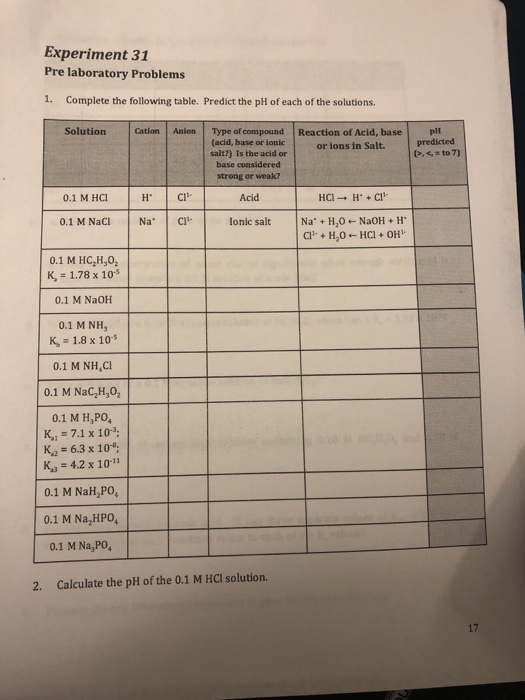please answer all We were unable to transcribe this image3. Calculate the pH, POH, [H') or (OH) for each of the solutions. [H,0" [OH] рон 59 4.7 x 10%M 9.0 x 10M 4. a. What is the molarity of pure water at 25°C? b. Does the concentration of water change significantly when enough acetic acid is added to water to make a 0.1 M solution of acetic acid? 5. What is the pH of a 0.10 M aqueous solution of...

• ### ____ 1. The diagram below represents serine, a polar, uncharged amino acid. Which functional group gives...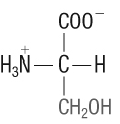____ 1. The diagram below represents serine, a polar, uncharged amino acid. Which functional group gives serine its distinct property? a. H3 b. CH2OH c. –H d. COO– ____ 2. The monomers shown below are monomers for which of the following natural polymers? a. polysaccharides b. plastics c. DNA d. proteins ____ 3. Which of the following processes illustrates the production of a protein? a. specific code for amino acids --> amino acid chain --> gene --> DNA --> specific...

• ### We were unable to transcribe this imageAn aqueous solution contains 0.444 M nitrous acid. Calculate the...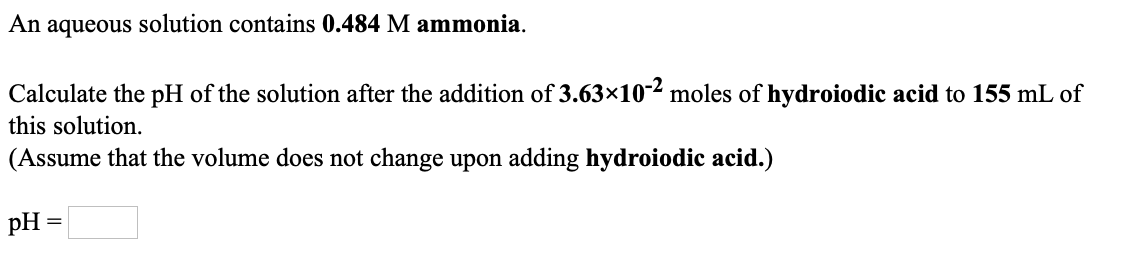We were unable to transcribe this imageAn aqueous solution contains 0.444 M nitrous acid. Calculate the pH of the solution after the addition of 3.02x10-2 moles of potassium hydroxide to 155 mL of this solution. (Assume that the volume does not change upon adding potassium hydroxide) pH = Acid Formula Kal Ка? | Каз Acetic acid CH3COOH 1.8x10-5 5.6x10-8 3.0x10-13 1.6x10-12 Acetylsalicylic acid (aspirin) HC9H704 3.0x10-4 Aluminum ion AI(H20)43+ 1.2x10-5 Arsenic acid H3As04 2.5*10-4 Ascorbic acid H2C6H606 7.9x10-5 Benzoic acid...

• ### Use the following information to answer questions 17-20. A large hospital uses a certain intravenous solution...Use the following information to answer questions 17-20. A large hospital uses a certain intravenous solution that it maintains in inventory. Assume the hospital uses reorder point method to control the inventory of this item. Pertinent data about this item are as follows: ------------------------------------------------------------ Forecast of demanda = 1,000 units per week Forecast errora, std. dev. =100 units per week Lead time = 4 weeks Carrying cost = 25 % per year Purchase price, delivered = \$52 per unit Replenishment...

• ### Cd2+ + Ni2++ 2H2O-Cd+NiO2+ 4H* In the above redox reaction, use oxidation numbers to identify the...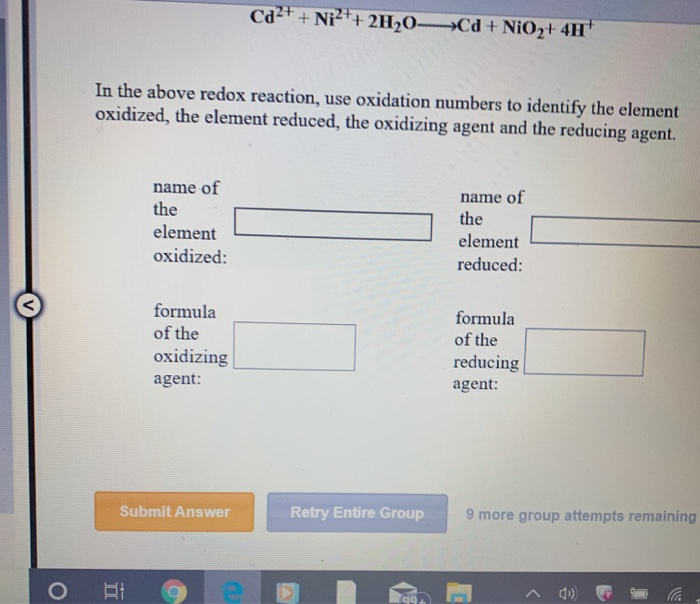Cd2+ + Ni2++ 2H2O-Cd+NiO2+ 4H* In the above redox reaction, use oxidation numbers to identify the element oxidized, the element reduced, the oxidizing agent and the reducing agent. name of the element oxidized: name of the element reduced: formula of the oxidizing agent: formula of the reducing agent: Submit Answer Retry Entire Group 9 more group attempts remaining о вое БТ Identify the species oxidized, the species reduced, the oxidizing agent a reducing agent in the following electron transfer reaction....

Free Homework App